# NCERT Solutions for Class 12 Chemistry Chapter 4 - Chemical Kinetics

The board exam preparation has been made easy with NCERT Solutions at Aasoka. Designed as per the latest CBSE syllabus, the solutions help students to score well on their boards as well as on competitive exams. Students preparing for competitive exams like NEET and JEE can easily do so with NCERT Solutions for Class 12. If students aspire to get good grades in order to follow their dream careers, then the solutions at Aasoka are the best way.

“Chemical Kinetics” of Class 12 Chemistry provides a good understanding of Arrhenius equation, collision theory of chemical reaction, rate of a reaction, factors affection rate of a reaction, dependence on the rate of reaction, rate of chemical reaction, and much more. In Chemistry, Chemical Kinetics is a branch that deals with the rate of the chemical reaction and its mechanism and factors affecting it.

##### Question 1:

From the concentration of C4H9Cl (butyl chloride) at different times given below, calculate the average rate of reaction: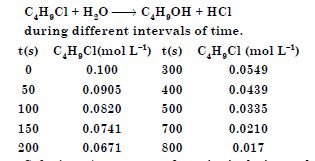Average rate of reaction in the interval t1 to t2 can be calculated as: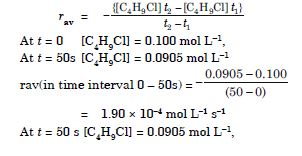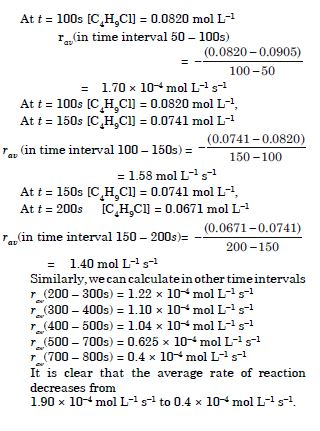##### Question 2:

Ammonia and oxygen react at high temperature as:
4NH3(g) + 5O2(g) ¾→ 4NO(g) + 6H2O (g)
In an experiment, rate of formation of NO is 3.6 × 10–3 mol L–1s–1. Calculate (i) rate of disappearance of ammonia, (ii) rate of formation of water.

For the reaction,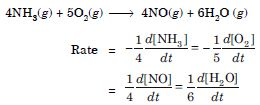(i) Rate of disappearance of ammonia
Rate of disappearance of NH3 = Rate of appearance of NO
= 3.6 × 10–3 mol L–1 s–1.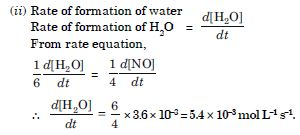##### Question 3:

Calculate the overall order of a reaction, which has the rate expression :
(i) Rate = k [A]1/2 [B]3/2 (ii) Rate = k [A]3/2 [B]–1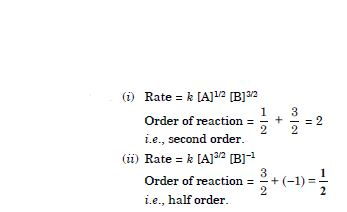##### Question 4:

Identify the reaction order from each of the following rate constants.

1. k = 2.3 × 10–5 L mol–1 s–1
2. k = 3.2 × 10–4 s–1

Order of a reaction can be predicted from the units of rate constant.

1. The unit of second order rate constant is L mol–1 s–1 and therefore, k = 2.3 × 10–5 L mol–1 s–1 represents a second order reaction.
2. The unit of a first order rate constant is s–1 and therefore, k = 3.2 × 10–4 s–1 represents a first order reaction.
##### Question 5:

The decomposition of N2O5 at 318 K according to the following equation follows first order reaction :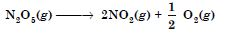The initial concentration of N2O5 was 1.24 × 10–2 mol L–1 and that after 60 minutes was 0.20 × 10–2 mol L–1. Calculate the rate constant of the reaction at 318 K.

For a first order reaction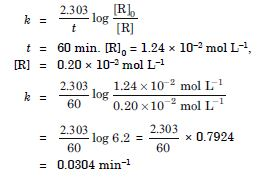##### Question 6:

The following data were obtained during the first order thermal decomposition of N2O5(g) at constant volume,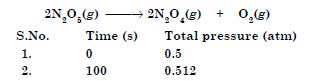Calculate the rate constant.

Let the pressure of N2O5 decreases by 2x atm. Since 2 moles of N2O5 decompose to give 2 moles of N2O4 and 1 mole of O2, then the pressure of N2O4(g) increases by 2x atm and that of O2(g) increases by x atm.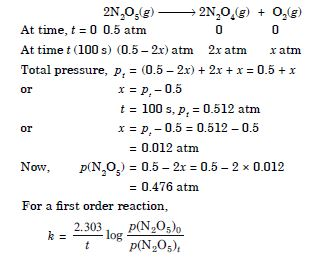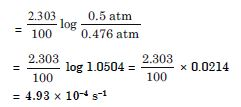##### Question 7:

A first order reaction is found to have a rate constant, k = 5.5 × 10–14 s–1. Find the half life period of the reaction.

For the first order reaction,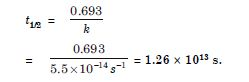##### Question 8:

Show that in case of a first order reaction, the time taken for completion of 99.9% reaction is ten times the time required for half change of the reaction.

When 99.9% reaction is completed,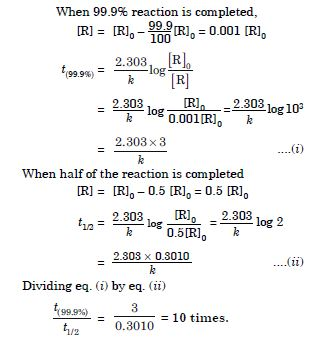##### Question 9:

The rate constant of a reaction at 500K and 700 K are 0.02 s–1 and 0.07 s–1 respectively. Calculate the values of Ea and A.

According to Arrhenius equation,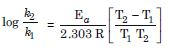k1 = 0.02 s–1, k2 = 0.07 s–1, T1 = 500 K, T2 = 700 K
Substituting the values :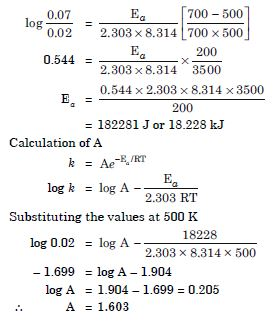##### Question 10:

The first order rate constant for the decomposition of ethyl iodide by the reaction:
C2H5I(g) $\to$ C2H4 (g) + HI (g)
at 600 K is 1.60 × 10–5 s–1. Its energy of activation is 209 kJ mol–1. Calculate the rate constant of the reaction at 700 K.

Ea = 209 kJ mol–1 = 209 × 103 J mol–1
k1 = 1.60 × 10–5 s–1,
T1 = 600 K
k2 = ? T2 = 700 K
Substituting the values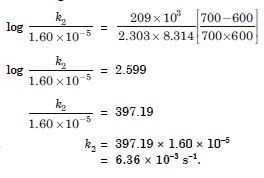##### Question 11:

In a reaction 2A $\to$ Products, the concentration of A decreases from 0.5 mol L–1 to 0.4 mol L–1 in 10 minutes. Calculate the rate during this time interval.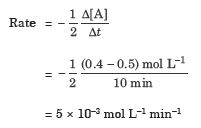##### Question 12:

For a reaction, A + B $\to$ Product, the rate is given by,
r = k[A]1/2[B]2.
What is the order of the reaction ?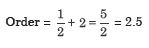##### Question 13:

The conversion of molecules X to Y follows second order kinetics. If concentration of X is increased three times how will it affect rate of formation of Y ?

Rate = k[A]2 or ka2 When conc. of A is increased 3 times, then [A] = 3a $\therefore$ Rate = k(3a)2 = k9a2 Rate of formation will become nine times.

##### Question 14:

A first order reaction has a rate constant 1.15 × 10–3 s–1. How long will 5g of this reactant take to reduce to 3g ?

For a first order reaction :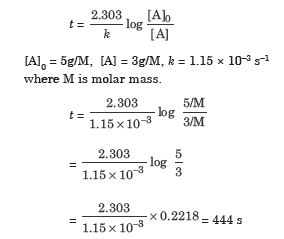##### Question 15:

Time required to decompose SO2Cl2 to half of its initial amount is 60 minutes. If the decomposition is a first order reaction. Calculate the rate constant of the reaction.

t1/2 = 60 min = 60 × 60 s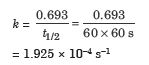##### Question 16:

What will be the effect of temperature on rate constant ?

The rate constant of a reaction increases with increase in temperature. In general, the rate constant becomes almost double with rise in temperature by 10$°$.

##### Question 17:

The rate of the chemical reaction doubles for an increase of 10 K in absolute temperature from 298 K. Calculate Ea.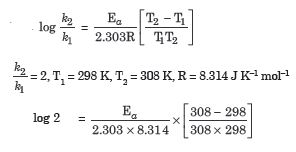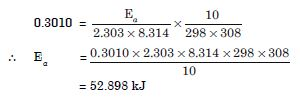##### Question 18:

The activation energy for the reaction
2HI (g) $\to$ H2(g) + I2(g)
is 209.5 kJ mol–1 at 581 K. Calculate the fraction of molecules of reactants having energy equal to or greater than activation energy.

The fraction of molecules having energy equal to or greater than activation energy,
Fraction of molecules = e– Ea/RT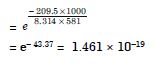##### Question 19:

From the rate expression for the following reactions, determine their order of reaction and the dimensions of the rate constants.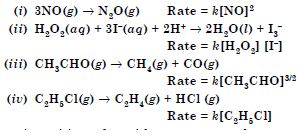The order with respect to each reactant and the overall order can be easily predicted from the rate equation.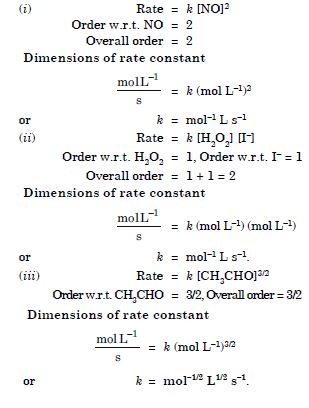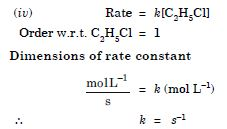##### Question 20:

For the reaction :
2A + B $\to$ 2AB
the rate = k[A][B]2 with k = 2.0 × 10–6 mol–2 L2 s–1. Calculate the initial rate of the reaction when [A] = 0.1 mol L–1, [B] = 0.2 mol L–1. Calculate the rate of reaction after [A] is reduced to 0.06 mol L–1.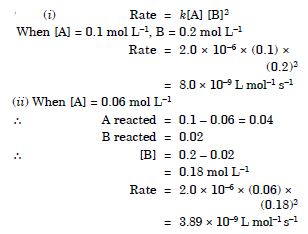##### Question 21:

The decomposition of NH3 on platinum surface is zero order reaction. What are the rates of production of N2 and H2 if k = 2.5 × 10–4 mol–1 L s–1.

2 NH3 $3}{4}$$3}{4}$ $\to$ N2 + 3 H2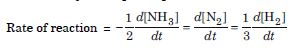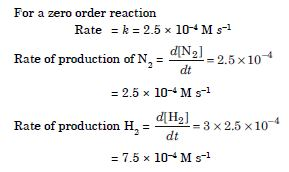##### Question 22:

The decomposition of dimethyl ether leads to the formation of CH4, H2 and CO and the reaction rate is given by
Rate = k[CH3OCH3]3/2
The rate of reaction is followed by increase in pressure in a closed vessel, so the rate can also be expressed in terms of the partial pressure of dimethyl ether, i.e., Rate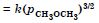If the pressure is measured in bar and time in minutes, then what are the units of rate and rate constants ?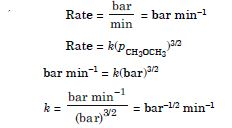##### Question 23:

Mention the factors that effect the rate of a chemical reaction.

There are a number of factors which influence the rate of a reaction. Some of the important factors are :

1. Concentration of the reactants. It has been observed that the rate of a chemical reaction keeps on decreasing with time. In other words, the rate of a reaction is directly proportional to the concentration of the reactants.
2. Temperature of the system. In general, an increase in the temperature increases the rate of almost all chemical reactions. On the other hand, a decrease in temperature decreases the rate. In general, the reaction rate for most of the chemical reactions becomes almost double, for every 10$°$ rise in temperature
3. Nature of reactants and the products. Rates of reactions are influenced by the nature of reactants and products. Since a chemical reaction involves the breaking of old bonds and the formation of new bonds, the reactivity of a substance can, therefore, be related to the ease with which the specific bonds are broken or formed and the number of such bonds involved.
4. Presence of catalyst. A catalyst, in general increases the rates of many reactions. For example, a mixture of H2 and O2 does not react at room temperature. However, in the presence of a catalyst such as finely divided platinum, the reaction becomes quite vigorous.
5. Surface area. The larger the surface area of the reactants, the faster is rate of reaction.
6. Exposure to radiation. In some cases, the rate of a chemical reaction is considerably increased by the use of certain radiations. The photons of these radiations having frequencies (n) possess sufficient energies (E = hn) to break certain bonds in reactants.
##### Question 24:

A reaction is second order with respect to a reactant. How is the rate of reaction affected if the concentration of the reactant is
(i) doubled (ii) reduced to half ?

Rate = k[A]2

1. When concentration of reactant is doubled, the rate becomes 4 times.
2. When concentration is reduced to 1/2, the rate becomes 1/4 times.
##### Question 25:

What is the effect of temperature on the rate constant of reaction ? How can this temperature effect on rate constant be represented quantitatively ?

Rate constant of a reaction increases with increase of temperature and becomes almost double for every 10$°$ rise in temperature. The effect of temperature on the rate constant is expressed quantitatively by Arrhenius equation,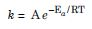where A is a constant of proportionality and is known as frequency factor, Ea is the activation energy, R is the gas constant and T is the absolute temperature. The frequency factor, A is related to the number of binary molecular collisions per second per litre.

##### Question 26:

In a pseudo first order hydrolysis of ester in water the following results were obtained :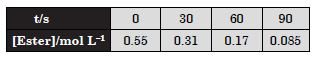Calculate the average rate of reaction between the time interval 30 to 60 seconds.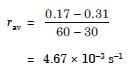##### Question 27:

A reaction is first order in A and second order in B.

1. Write the differential rate equation
2. How is the rate affected on increasing the concentration of B three times ?
3. How is the rate affected when the concentrations of both A and B are doubled ?
1. Rate = k[A] [B]2
2. Rate becomes 9 times
3. Rate becomes 8 times
##### Question 28:

In a reaction between A and B, the initial rate of reaction (r0) was measured for different initial concentrations of A and B as given below :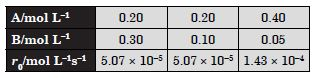What is the order of the reaction with respect to A and B ?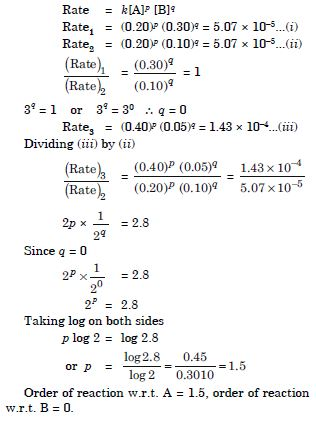##### Question 29:

The following results have been obtained during the kinetic studies of the reaction :
2A + B $\to$ C + D

Determine the rate law and the rate constant for the reaction.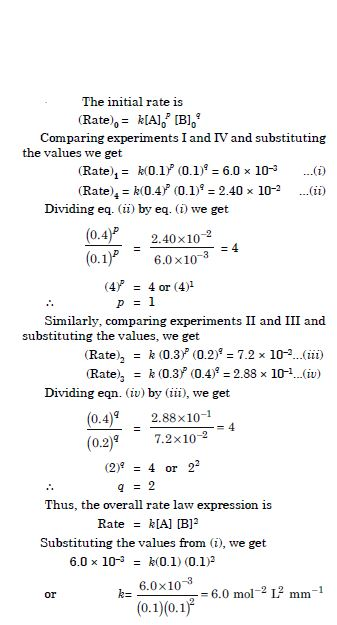##### Question 30:

The reaction between A and B is first order with respect to A and zero order with respect to B.
Fill in the blanks in the following table :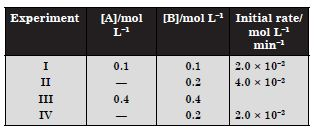Rate = k[A][B]0
2.0 × 10–2 = k [0.1] or k = 0.2 min–1
2nd line :
4.0 × 10–2 = 0.2 [A]
or [A] = 0.2 mol L–1
3rd line : Rate = 0.2 × 0.4
= 8.0 × 10–2 mol L–1 min–1
4th line :
2.0 × 10–2 = 0.2[A] or [A] = 0.1 mol L–1

##### Question 31:

Calculate the half-life of a first order reaction from their rate constants given below:
(i) 200 s–1 (ii) 2 min–1 (iii) 4 years–1

For the first order reaction,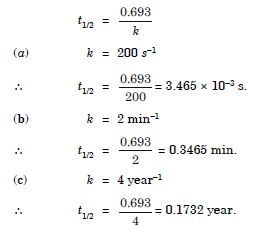##### Question 32:

The half-life for radioactive decay of14C is 5730 years. An archaeological artifact contained wood had only 80% of the 14C found in a living trees. Estimate the age of the sample.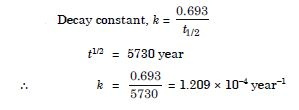Since the rate of counts is proportional to the number of 14C atoms in the sample,
N0 = 100, N = 80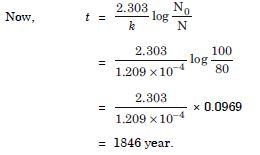##### Question 33:

The experimental data for decom-position of N2O5
2N2O5 $3}{4}$$3}{4}$ $\to$ 4NO2 + O2
in gas phase at 318K are given below :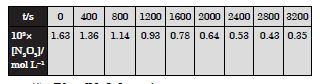1. Plot [N2O5] against t.
2. Find the half-life period for the reaction.
3. Draw a graph between log [N2O5] and t.
4. What is the rate law ?
5. Calculate the rate constant.
6. Calculate the half-life period from k and compare it with (b).

(i) The plot of [N2O5] versus time is shown below :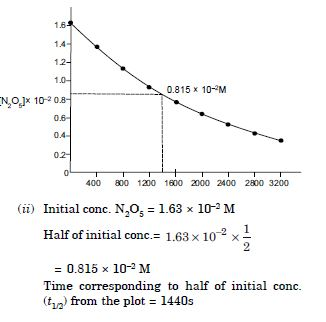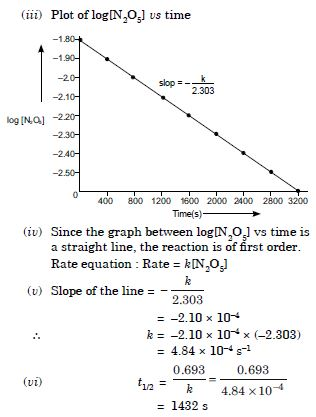##### Question 34:

The rate constant for a first order reaction is 60 s–1. How much time will it take to reduce the initial concentration of the reactant to its 1/16th value ?

Let initial concentration, [A]0 = a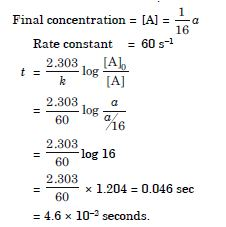##### Question 35:

During nuclear explosion, one of the products is 90Sr with half-life of 28.1 years. If 1 $\mathrm{\mu }$g of 90Sr was absorbed in the bones of a newly born baby instead of calcium. How much of it will remain after 10 years and 60 years if it is not lost metabolically?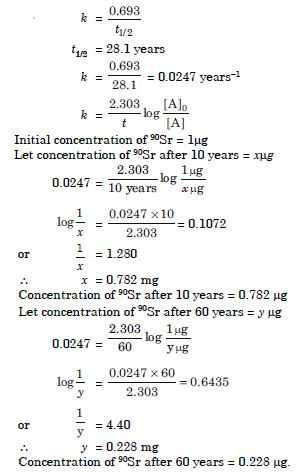##### Question 36:

Show that time required for 99% completion is twice the time required for the completion of 90% reaction.

For a first order reaction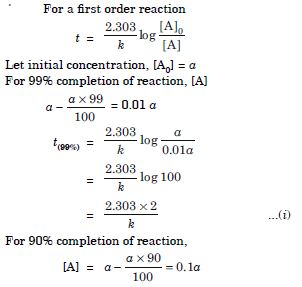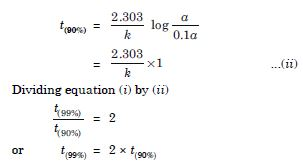##### Question 37:

A first order reaction takes 40 min for 30% decomposition. Calculate t1/2.

Let initial conc. = a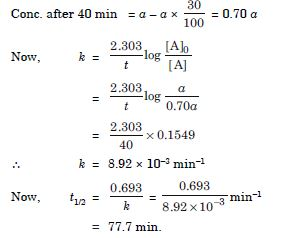##### Question 38:

For the decomposition of azoisopropane to hexane and nitrogen at 543 K, the following data is obtained.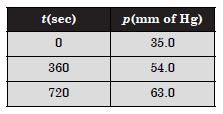Calculate the rate constant.

Let initial pressure of azoisopropane = pi
Let x atm of azoisopropane decreases at time t so that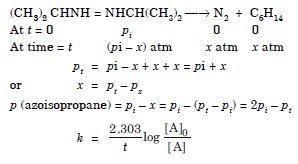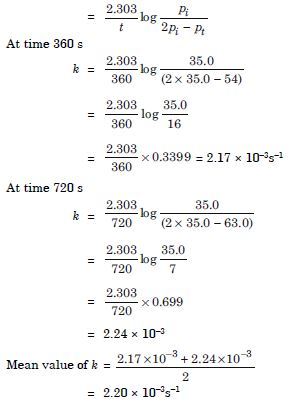##### Question 39:

The following data were obtained during the first order thermal decomposition of SO2Cl2 at a constant volume.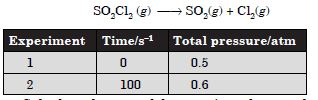Calculate the rate of the reaction when total pressure is 0.65 atm.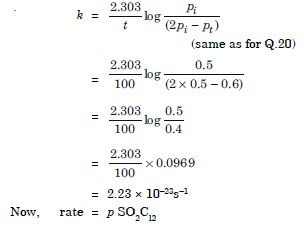Pressure of SO2Cl2 when total pressure = 0.65 atm
pSO2Cl2 = 2pi – pt = 2 × 0.5 – 0.65 = 0.35 atm
$\therefore$ Rate = 2.23 × 10–3 × 0.35 = 7.805 × 10–4 atm s–1

##### Question 40:

The rate constant for the decomposition of hydrocarbons is 2.148 × 10–5 s–1 at 546 K. If the energy of activation is 179.9 kJ/mol. What will be the value of pre-exponential factor?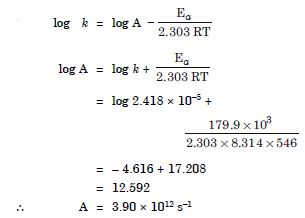##### Question 41:

Consider a certain reaction A $\to$ Products with k = 2.0 × 10–2s–1. Calculate the concentration of A remaining after 100 s if the initial concentration of A is 1.0 mol L–1.

k = 2.0 × 10–2 s–1
t1 = 0, [A]0 = 1.0 M
t2 = 100s, [A] = ?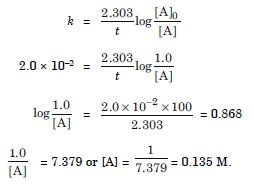##### Question 42:

Sucrose decomposes in acid solution into glucose and fructose according to the first order rate law, with t1/2 = 3.00 hours. What fraction of sample of sucrose remains after 8 hours?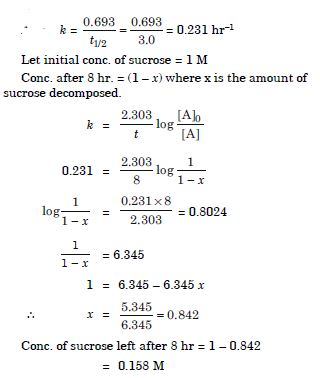##### Question 43:

The decomposition of hydrocarbon follows the equation k = (4.5 × 1011s–1)e–28000K/T. Calculate Ea.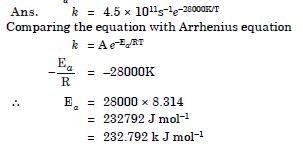##### Question 44:

The rate constant for the first order decomposition of H2O2 is given by the following equation :
log k = 14.34 – 1.25 × 104K/T
Calculate Ea for this reaction and at what temperature will its half-period be 256 minutes?

log k = 14.34 – 1.25 × 104K/T ...(i)
Comparing with equation,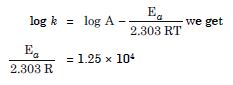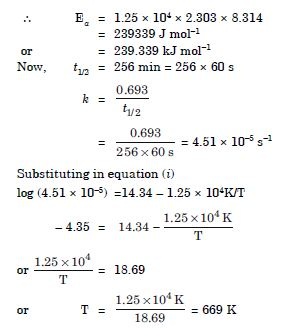##### Question 45:

The decomposition of A product has value of k as 4.5 × 103 s–1 at 10$°$C and energy of activation 60 kJ mol–1. At what temperature would k be 1.5 × 104 s–1?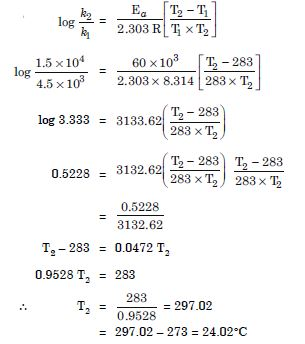##### Question 46:

The time required for 10% completion of a first order reaction at 298 K is equal to that required for its 25% completion at 308 K. If the value of A is 4 × 1010s–1, calculate k at 318 K and Ea.

For 10% completion of the reaction,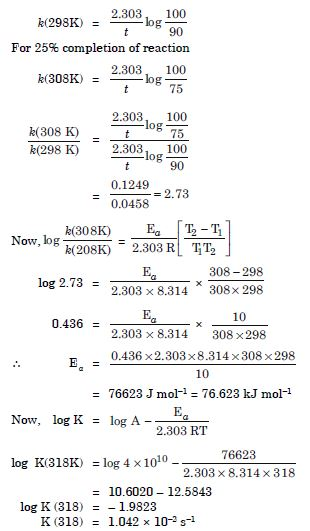##### Question 47:

The rate of reaction quadruples when the temperature changes from 293 K to 313 K.
Calculate the energy of activation of the reaction assuming that it does not change with temperature.

T1 = 293 K, T2 = 313 K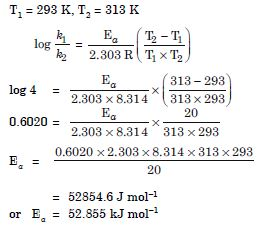##### Question 48:

State a condition under which a bimolecular reaction is kinetically first order reaction.

Bimolecular reaction becomes kinetically first order when one of the reactants is in excess.

##### Question 49:

Write the rate equation for the reaction
2A + B $\to$ C if the order of the reaction is zero.

Rate = k [A]0[B]0 or Rate = k

##### Question 50:

How can you determine the rate law of the following reaction?
2NO(g) + O2(g) $\to$ 2NO2 (g)

The rate law can be determined by measuring the rate of this reaction as a function of initial concentration by keeping the concentration of one of the reactants constant and changing the concentration of other reactant or by changing the concentration of both the reactants. From the concentration dependance of rate, rate law can be determined.

##### Question 51:

For which type of reactions, order and molecularity have the same value?

For elementary reaction, order and molecularity have the same value.

##### Question 52:

In a reaction if the concentration of reactant A is tripled, the rate of reaction becomes twenty seven times. What is the order of the reaction?

Order is 3 because
k = [A]3

##### Question 53:

Derive an expression to calculate time required for completion of zero order reaction.

For a zero order reaction,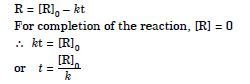##### Question 54:

For a reaction A + B $\to$ Products, the rate law is : Rate = k[A][B]3/2.
Can the reaction be an elementary reaction? Explain.

The reaction is not elementary because order of the reaction is fractional 1 +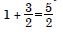##### Question 55:

For a certain reaction large fraction of molecules has energy more than the threshold energy, yet the rate of reaction is very slow. Why?

Though the reacting molecules may be having energy more than threshold energy, yet they may not be effective due to lack of proper orientation.

##### Question 56:

For a zero order reaction will the molecularity be equal to zero? Explain.

Molecularity can never be zero or a fractional number.

##### Question 57:

For a general reaction A $3}{4}$$3}{4}$$\to$ B, plot of concentration of A vs time is given in figure below. Answer the following question on the basis of this graph.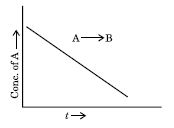1. What is the order of the reaction?
2. What is the slope of the curve?
3. What are the units of rate constant?
1. zero order reaction
2. [R] = [R0] – kt
$\therefore$ Slope = – k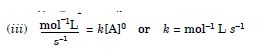##### Question 58:

The reaction between H2(g) and O2(g) is highly feasible yet allowing the gases to stand at room temperature in the same vessel does not lead to the formation of water. Explain.

This is because activation energy for the reaction is very high at room temperature.

##### Question 59:

Why does the rate of a reaction increase with rise in temperature?

At higher temperatures, larger fraction of colliding particles can cross the energy barrier (i.e. the activation energy), which leads to faster rate. For detail refer text.

##### Question 60:

Oxygen is available in plenty in air yet fuels do not burn by themselves at room temperature. Explain.

The activation energy for combustion reactions of fuels is very high at room temperature therefore they do not burn by themselves.

##### Question 61:

Why is the probability of reaction with molecularity higher than three very rare?

The probability of more than three molecules colliding simultaneously is very small. Therefore, the possibility of molecularity being three is very low.

##### Question 62:

Why does the rate of any reaction generally decreases during the course of the reaction?

The rate of a reaction depends on the concentration of reactants. As the reaction progresses, the concentration of reactant decreases because the reactants start getting converted to products. Hence the rate decreases.

##### Question 63:

Thermodynamic feasibility of the reaction alone cannot decide the rate of the reaction. Explain with the help of one example.

Thermodynamic feasibility (–ve $∆$G) cannot alone decide the rate of reaction because the reaction may be feasible but its rate may be very very slow because of very high activation energy. For example, conversion of diamond to graphite is highly feasible but this reaction is very slow because it has high activation energy.

##### Question 64:

Why in the redox titration of KMnO4 vs oxalic acid, we heat oxalic acid solution before starting the titration?

The reaction between KMnO4 and oxalic acid is very slow. By raising the temperature we can increase the rate of reaction.

##### Question 65:

Why can’t molecularity of any reaction be equal to zero?

Molecularity is the number of molecules taking part in an elementary step. For this we require at least a single molecule leading to the value of minimum molecularity of one.

##### Question 66:

Why molecularity is applicable only for elementary reactions and order is applicable for elementary as well as complex reactions?

KClO3 + 6FeSO4 + 3H2SO4 $\to$ KCl + 3H2O + 3Fe2(SO4)3